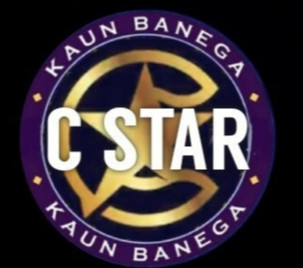# Kaun Banega C-star (Set 1)

9 Questions | Total Attempts: 15SettingsCreate your own Quiz• 1.
1) Which among the below is not a data type associated with C ?
• A.

Int

• B.

Float

• C.

Object

• D.

Void

• 2.
2) All keywords in C are _____________.
• A.

Lowercase letters

• B.

Uppercase letters

• C.

Camelcase letters

• D.

Both a & b

• 3.
3) Which among the following is odd one out?
• A.

Printf()

• B.

Scanf()

• C.

Putchar()

• D.

Fprintf()

• 4.
4) What is the size of int data type ?
• A.

4 Bytes

• B.

8 Bytes

• C.

Depends on the system/ compiler

• D.

2 Bytes

• 5.
5) Which of the following declarations is not supported in c?
• A.

String str

• B.

Char *str

• C.

Float str=3e2

• D.

Both a and c

• 6.
6) For a typical program input is taken using ____________
• A.

Scanf

• B.

Files

• C.

Command line

• D.

All of the above

• 7.
7) Point out the error (if any) in the following C code? #include<stdio.h> enum hello {     a,b,c; }; main() {     enum hello m;     printf("%d",m); }
• A.

No error

• B.

Error in the statement: a,b,c;

• C.

Error in the statement: enum hello m;

• D.

Error in the statement: printf(“%d”,m);

• 8.
8) In C language, FILE is of which data type?
• A.

Int

• B.

Char*

• C.

Struct

• D.

None of the mentioned

• 9.
9) What will be the output of the following code? #include<stdio.h> #define square(x) x*x void main() { int i; i=64/square(4); printf(“%d”,i); }
• A.

4

• B.

64

• C.

16

• D.

None of these

Related TopicsBack to top
×

Wait!
Here's an interesting quiz for you.